# CreatingConnect CurvesThis task shows how to create a connecting curve between two curves.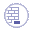Open the ConnectCurve1.CATPart document.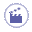1. Click Connect Curve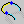.

 The Connect Curve Definition dialog box appears.
2. Select the Connect type.

## Normal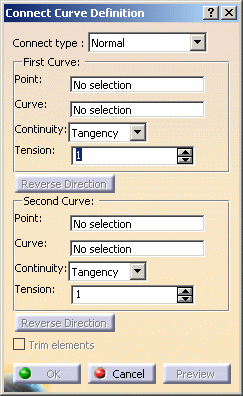• Select a first Point on a curve then a second Point on a second curve.
The Curve fields are automatically filled.

## Base Curve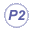This type is only available with the Generative Shape Design 2 product.
The Base Curve option is useful when creating several profiles or guides that have the same shape.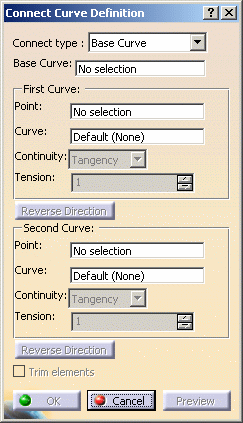• Select a base curve as the curve reference.
The orientation of the connect curve will be the orientation of the base curve.
• Select a first Point on a curve then a second Point on a second curve.
It can be either on the base curve or on the support curve.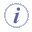Selecting the support curve is optional (it is set as Default).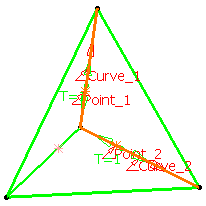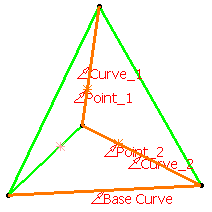Normal curve Base Curve
3. Use the drop-down lists to specify the desired Continuity type: Point, Tangency or Curvature.

4. If needed, enter tension values.

 A graphic manipulator also allows you to modify the tension at the extremity of the connect curve, rather than in the dialog box.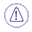If the Base Curve type is selected, the Continuity and Tension options for the first and the second curve are grayed out and set to Tangency and 1 respectively. The connect curve is displayed between the two selected points according to the specified continuity and tension values.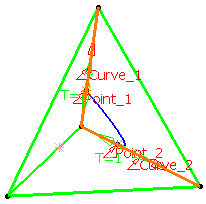Normal curve with point continuity at both points Both tensions = 1 Normal curve with point continuity at first point and tangent continuity at the second point Both tensions = 1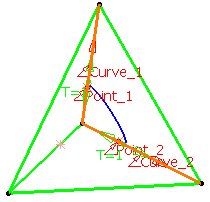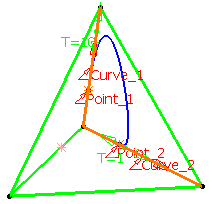Normal curve with tangent continuity at first point and curvature continuity at the second point Both tensions = 1 Normal curve with tangent continuity at first point and curvature continuity at the second point First tension = 10 and second tension = 1A red arrow is displayed at each extremity of the curve: the orientation of the arrow of the first/second direction corresponds to that of the connect curve's tangent to the first/second selected point.
5. Click the arrow to reverse the orientation of the curve at that extremity or click Reverse Direction.If the Base Curve type is selected, the Reverse Direction buttons are grayed out.
6. Check the Trim elements option if you want to trim and assemble the two initial curves to the connect curve.If the Base Curve type is selected, you must select a support curve to access the Trim elements option. Otherwise, it is grayed out.
7. Click OK to create the connect curve.

 The curve (identified as Connect.xxx) is added to the specification tree.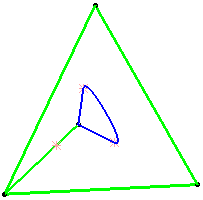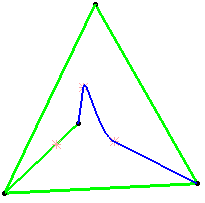Normal curve with tangent continuity at both points Both tensions = 1 Base Curve Trim elements option checked